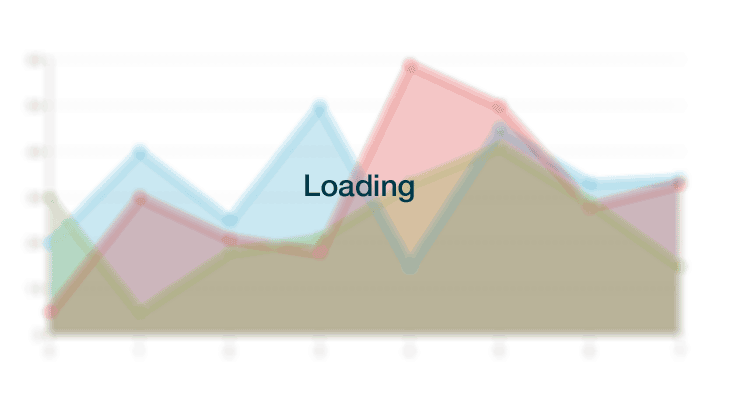Show Nav

JavaScript Pareto Charts

Pareto Chart Overview

Pareto charts display both a bar and a line graph, where individual values are represented in descending order by bars, and the cumulative total is represented by the line. The left vertical axis is the frequency of occurrence, but it can alternatively represent cost or another important unit of measure. The right vertical axis is the cumulative percentage of the total number of occurrences, total cost, or total of the particular unit of measure. ZingChart allows for multiple styling options of JavaScript pareto charts.```{
"type": "pareto",
"series": [
{
"values": [4642, 4345, 2350, 1251]
}
]
}```

Data Format

Value Array

Create a "series" object array of length 1. Within the object, place a "values" array of numbers. ZingChart will automatically calculate the percentage values for the line plot from this array.

```{
"type":"pareto",
"series":[
{
"values":[464200,434500,235000,125100]
}
]
}```

Pareto Chart-specific Properties

Pareto charts include a number of unique chart-specific properties that allow you to manipulate the appearance and behaviors of your HTML5 pareto chart.

Pareto Line Plot

The “line-plot” object sets the definition for the auto-generated line plot.

```{
"type":"pareto",
"options":{
"line-plot":{
"line-color":"#f00",
"line-width":4
}
},
"series": [
{
"values": [4642, 4345, 2350, 1251]
}
]
}```Scale-Y-2

The “scale-y-2” object sets the definition for the second Y scale (with percentages as values), the one associated with the auto-generated line plot.

```{
"type":"pareto",
"options":{
"scale-y-2":{
"line-color":"red",
"label":{
"text":"Percentage",
"font-color":"red"
},
"tick":{
"line-color":"red"
},
"item":{
"color":"red"
}
}
},
"plotarea":{
"margin-right":"10%"
},
"series": [
{
"values": [4642, 4345, 2350, 1251]
}
]
}```